# Calculus Differentiation Calculus Differentiation dydx y Calculus Differentiation

• Slides: 29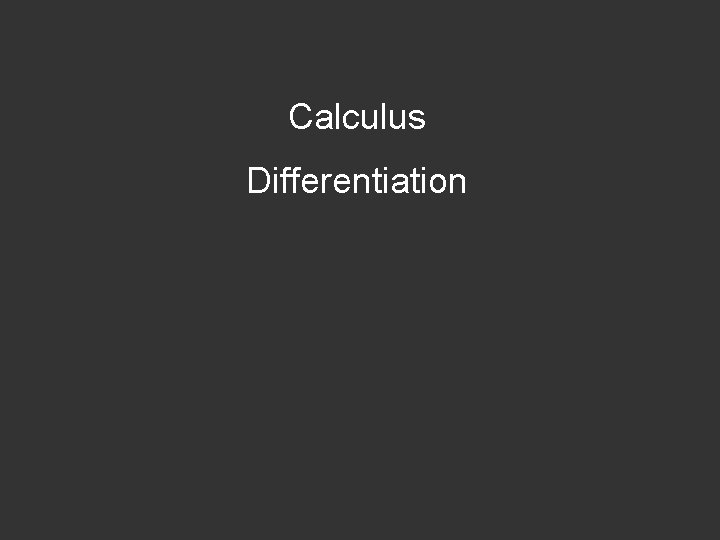Calculus Differentiation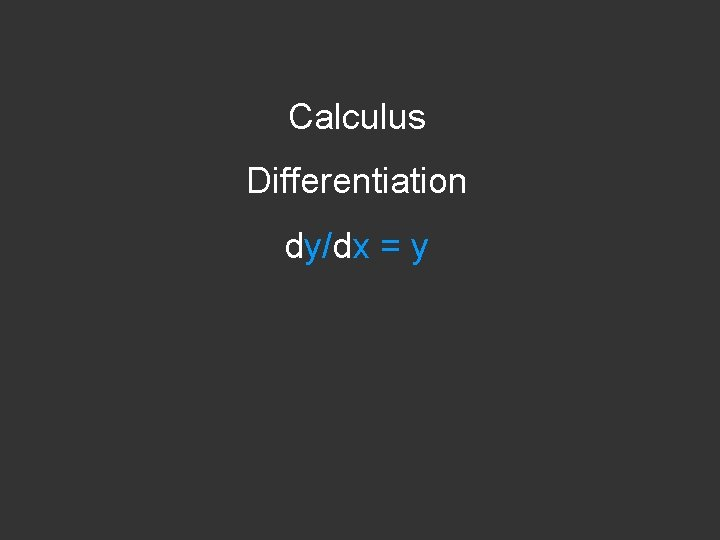Calculus Differentiation dy/dx = y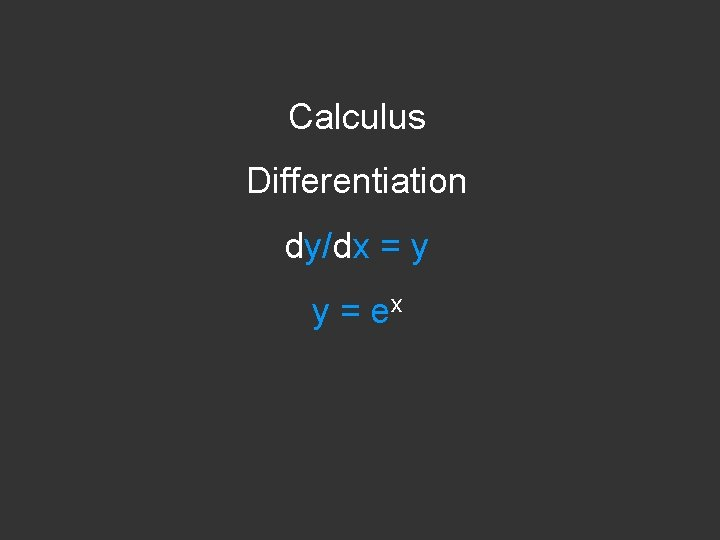Calculus Differentiation dy/dx = y y = exCalculus Differentiation dy/dx = y y = ex eix = cosx + i sinxdsinx/dx = cosx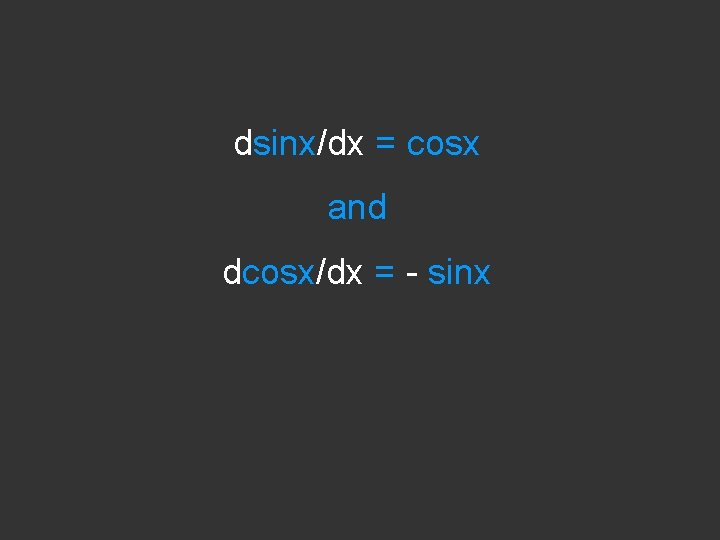dsinx/dx = cosx and dcosx/dx = - sinxdsinx/dx = cosx and dcosx/dx = - sinx thus d 2 sinx/dx 2 = -sinx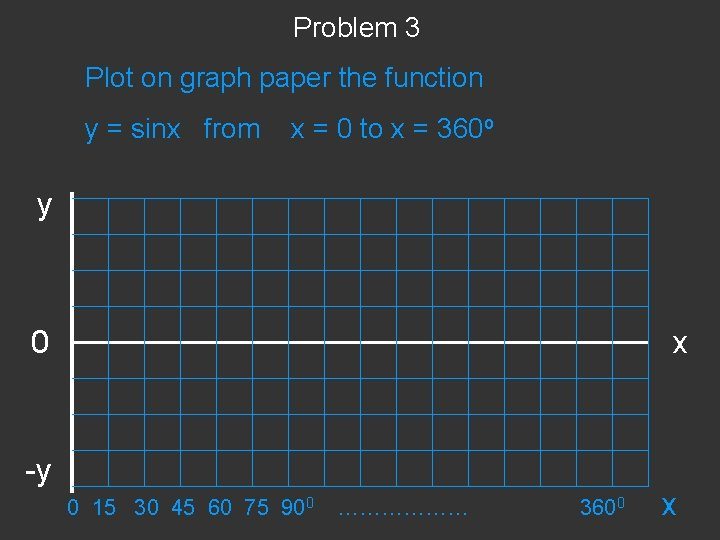Problem 3 Plot on graph paper the function y = sinx from x = 0 to x = 360 o y 0 -y x 0 15 30 45 60 75 900 ……………… 3600 xProblem 3 Plot on graph paper the function y = sinx from x = 0 to x = 360 o y 0 -y x 0 15 30 45 60 75 900 ……………… 3600 x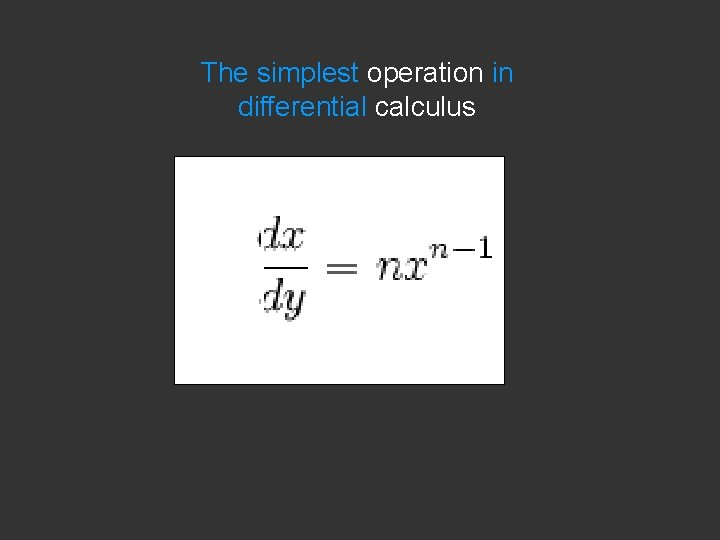The simplest operation in differential calculus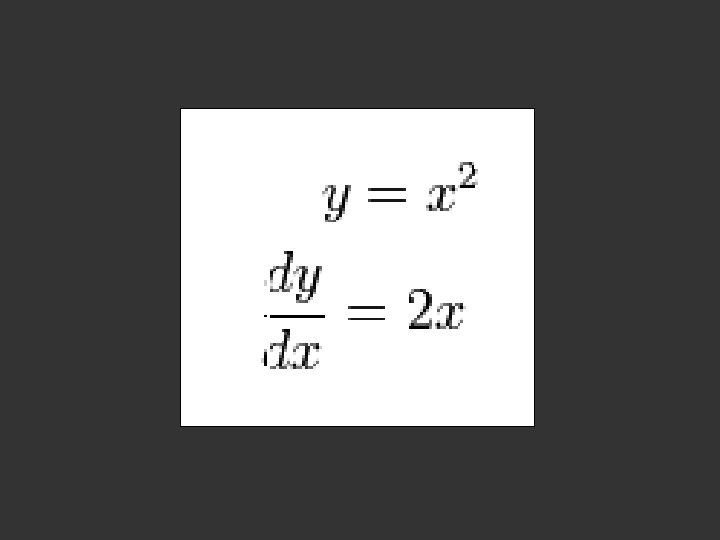When you see e ……. think WAVES x e at: thestewscope. wordpress. com/2009/07/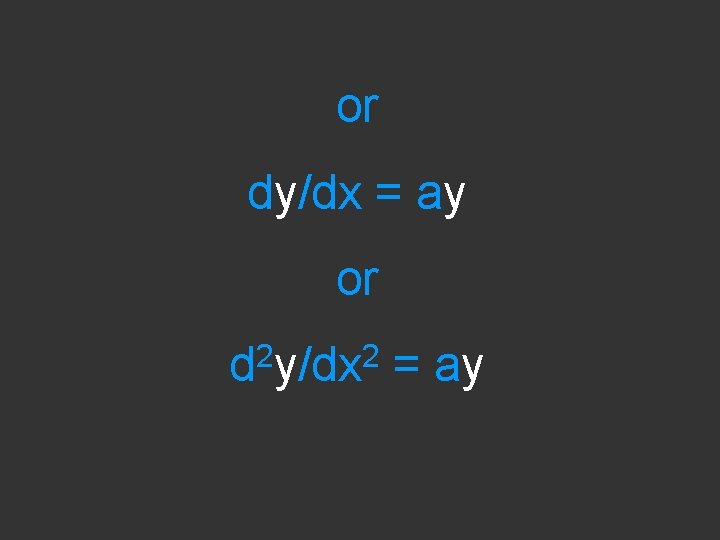or dy/dx = ay or 2 2 d y/dx = ayat: zaksiddons. wordpress. com/. . . /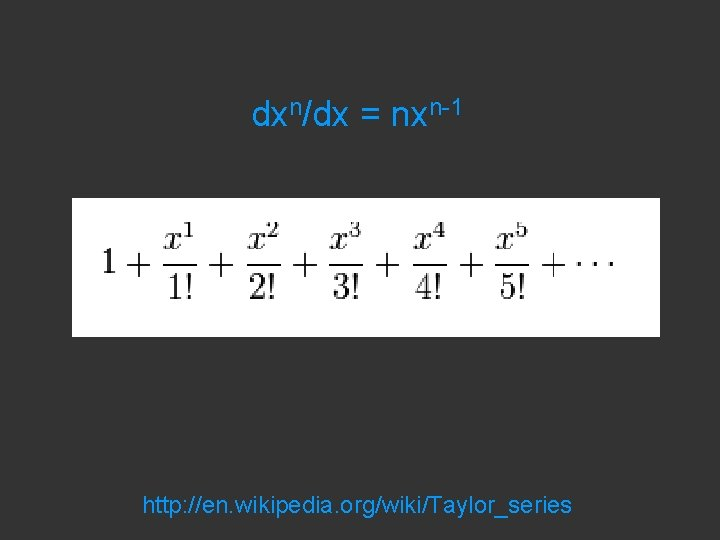dxn/dx = nxn-1 http: //en. wikipedia. org/wiki/Taylor_series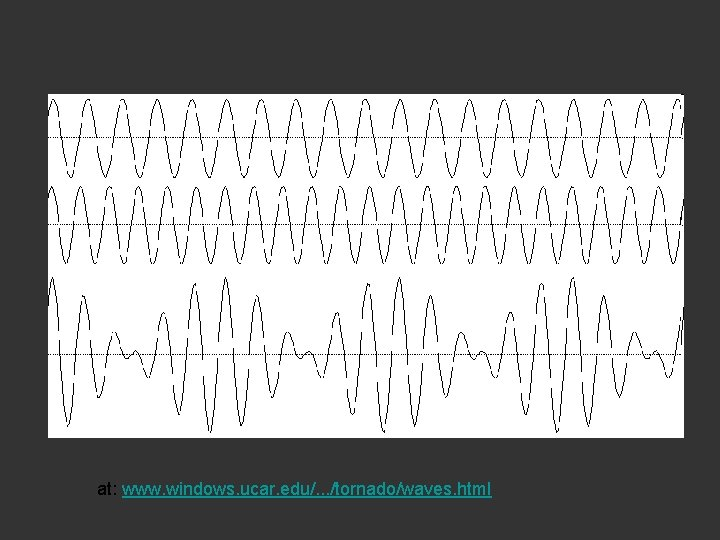at: www. windows. ucar. edu/. . . /tornado/waves. htmlimage at: www. physics. utoronto. ca/. . . /lec 05/index. htm e at: thestewscope. wordpress. com/2009/07/The Taylor series for the exponential function ex at a = 0 is The above expansion holds because the derivative of ex with respect to x is also ex and e 0 equals 1. This leaves the terms (x − 0)n in the numerator and n! in the denominator for each term in the infinite sum. dxn/dx = nxn-1 http: //en. wikipedia. org/wiki/Taylor_series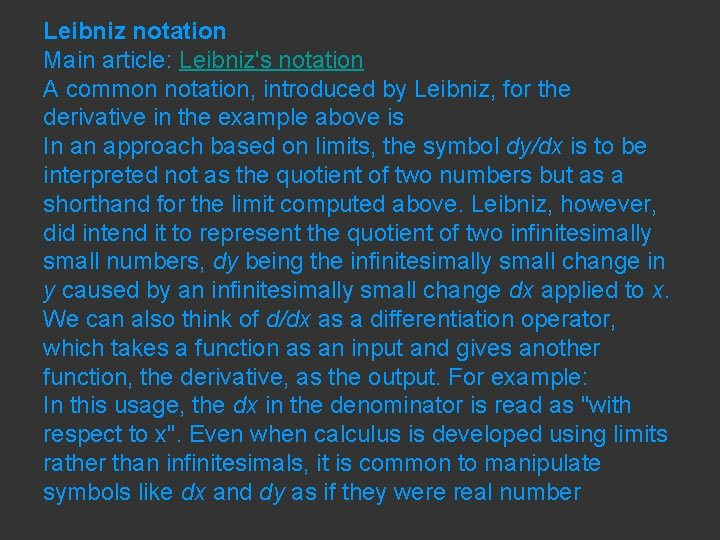Leibniz notation Main article: Leibniz's notation A common notation, introduced by Leibniz, for the derivative in the example above is In an approach based on limits, the symbol dy/dx is to be interpreted not as the quotient of two numbers but as a shorthand for the limit computed above. Leibniz, however, did intend it to represent the quotient of two infinitesimally small numbers, dy being the infinitesimally small change in y caused by an infinitesimally small change dx applied to x. We can also think of d/dx as a differentiation operator, which takes a function as an input and gives another function, the derivative, as the output. For example: In this usage, the dx in the denominator is read as "with respect to x". Even when calculus is developed using limits rather than infinitesimals, it is common to manipulate symbols like dx and dy as if they were real numberMain article: Leibniz's notation A common notation, introduced by Leibniz, for the derivative in the example above is In an approach based on limits, the symbol dy/dx is to be interpreted not as the quotient of two numbers but as a shorthand for the limit computed above. Leibniz, however, did intend it to represent the quotient of two infinitesimally small numbers, dy being the infinitesimally small change in y caused by an infinitesimally small change dx applied to x. We can also think of d/dx as a differentiation operator, which takes a function as an input and gives another function, the derivative, as the output. For example:Problem 3 Plot on graph paper the function y = sinx from x = 0 to x = 360 o y 0 -y x 0 15 30 45 60 75 900 ……………… 3600 x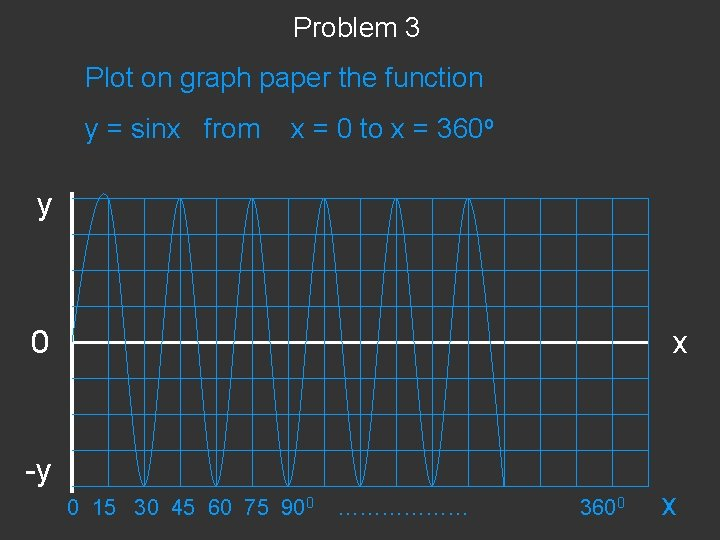Problem 3 Plot on graph paper the function y = sinx from x = 0 to x = 360 o y 0 -y x 0 15 30 45 60 75 900 ……………… 3600 x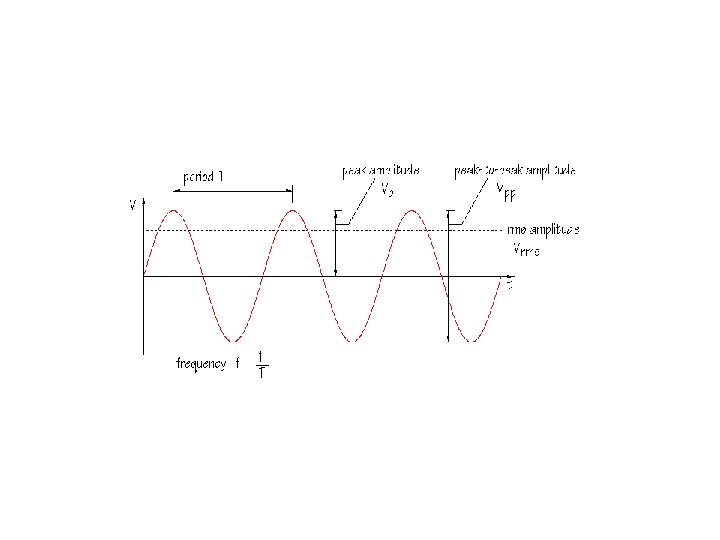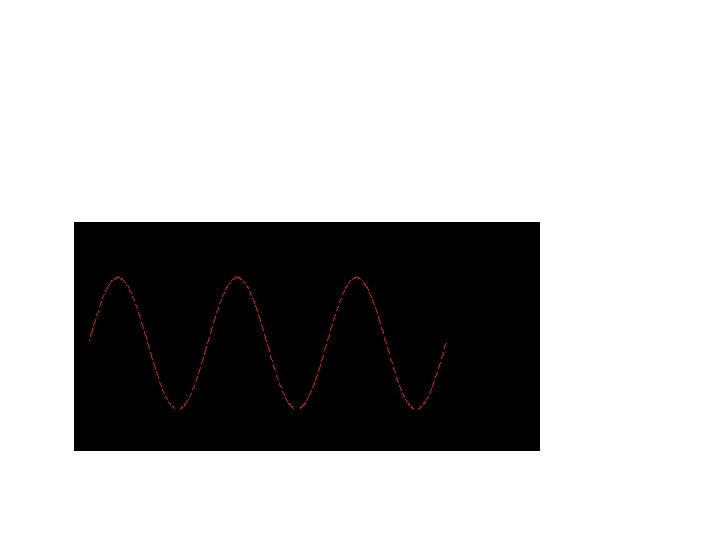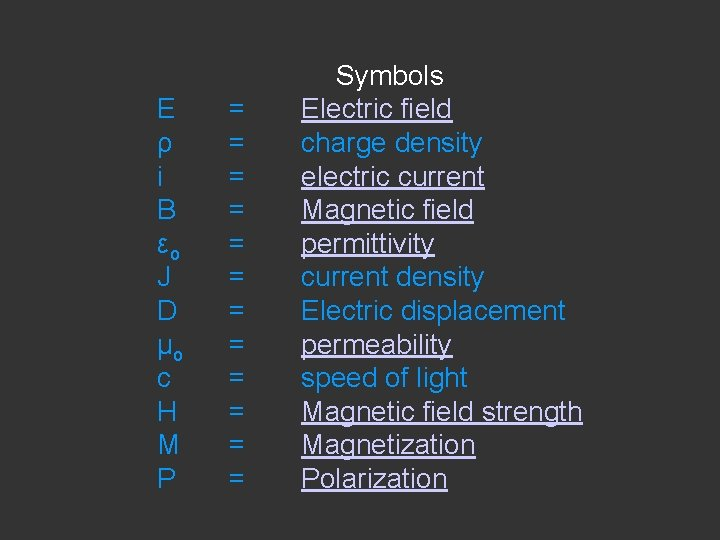E ρ i B ε o J D μ o c H M P = = = Symbols Electric field charge density electric current Magnetic field permittivity current density Electric displacement permeability speed of light Magnetic field strength Magnetization Polarization